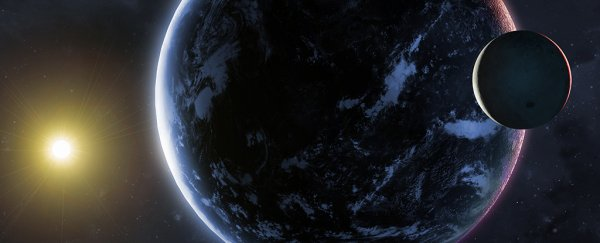(Mark Garlick/Science Photo Library/iStock)

# Scientists Say They've Found a Way to Solve The 'Oldest Open Question in Astrophysics'

29 DECEMBER 2019

After almost 350 years, physicists have just arrived at a statistical solution for Newton's three-body problem – that is, the problem of figuring out how three similar objects or bodies are going to travel in space in a way that fits in with the laws of motion and gravity.

The researchers behind the latest study describe the three-body problem as "arguably the oldest open question in astrophysics", and while they haven't completely cracked the case, they've gotten closer than most by finding a statistical formula that fits this open question in certain scenarios.

In particular, they looked at a couple of centuries of previous research that puts forward the following idea: in unstable, chaotic three-body systems, one of those bodies eventually gets expelled, leaving behind a stable binary relationship between the two that are left.

While the researchers point out that they haven't come up with an exact, complete solution for the three-body problem, they have developed a working statistical method that covers a lot of these three-body to two-body events, one which can be very useful in helping physicists visualise complicated processes.

"When we compared our predictions to computer-generated models of their actual movements, we found a high degree of accuracy," says astrophysicist Nicholas Stone, from the Hebrew University of Jerusalem.

The three laws of motion laid down by Isaac Newton in 1687 are these: that objects remain in a state of inertia unless acted upon by force, that the relationship between acceleration and applied force is force equals mass times acceleration (F=ma), and that for every action there is an equal and opposite reaction.

So far so brilliant, as far as the basic physics of the Universe are concerned. But Newton ran into difficulties applying his rules to the Earth, Moon and Sun – the original three bodies. It actually became much harder to track three bodies with these mathematical rules.

While scientists have found fixes for special cases, a general formula for the three-body problem has proved elusive. It's like trying to apply a mathematical template to the butterfly effect – it's just too chaotic to track.

In this case, the researchers applied a probability hypothesis called ergodicity to help guide them to their answers, which uses the principles of averages to work out what's going to happen in a particular system. Importantly, over time, ergodic processes bear little relation to their original state (just like a three-body system).

What the new solution does is give scientists an understanding of how the two survivors of a three-body problem are going to behave in a variety of newly stable scenarios – and that sort of understanding can be crucial in astrophysics.

"Take three black holes that are orbiting one another," says Stone. "Their orbits will necessarily become unstable and even after one of them gets kicked out, we're still very interested in the relationship between the surviving black holes."

The research has been published in Nature.